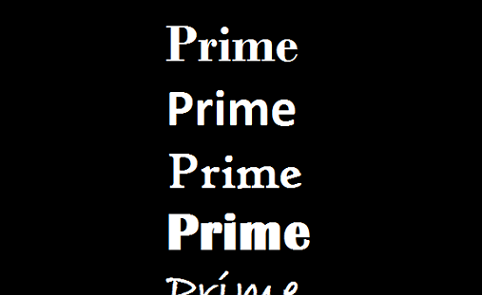# Prime Generating Function 2Find the smallest positive integer $n$ for which the function $f(n)=3n^2+3n+23$ is composite.

×